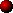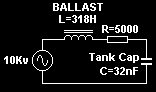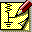COMPUTER BASED SIMULATION

Although Tesla coils are relatively basic systems in terms of component count, their operation is often far from simple. In each stage of the Tesla coil system there are quite complex interactions caused by only a few components.

In the charging circuit the connection together of the ballast inductor and tank capacitor creates a resonant circuit. The behaviour of this LC circuit is familiar to many in the frequency domain, and its steady state characteristics are also well defined by mathematics. However, when a spark gap is added to the charging system the analysis becomes more complicated. We are now faced with a system which is resonant and contains a non-linear switching component ! What is more, we are often interested in voltages and currents with respect to time, rather than with respect to frequency. Add to this effects such as inductive-kick and saturation, and it becomes obvious that a mathematical analysis of even the simplest of charging circuits is not so simple at all.

The Primary and Secondary coils present a similar situation. In isolation , the primary and secondary circuit behaviours can be represented by simple differential equations. However, when the two coils are brought together, they interact due to magnetic coupling and the mathematics instantly takes a turn for the worse.

The behaviour of these systems can actually be represented very accurately by mathematics. The problem is that the number and complexity of the calculations makes manual analysis quite difficult. The solution is to use a computer program designed specifically for this purpose.

The PSpice simulator package produced by Microsim is a piece of software which works as follows:

1. You draw an electrical schematic of the system which you want to study,

2. The computer package converts the schematic into a mathematical model,

3. The computer rapidly calculates the complex simultaneous equations that model your circuit,

4. The computer converts the results of the maths back into voltages, and currents which relate to the schematic,

5. Any voltage, current, power waveform etc. can be requested and will be displayed on screen.

A typical Tesla coil system is actually relatively easy for a simulation package such as Microsim to process. Usually the computer will take around 20 seconds to do all of the calculations and present the results !

The advantages of computer based simulation for the Tesla Coil designer are numerous:

1. Simulation allows quick evaluation of different ideas. For example, the effects of different capacitor sizes or different rotary speeds can be evaluated in a few minutes without risk of damaging any real components. Gut feelings are OK, but running something through a simulator first can highlight potentially costly oversights.

2. Simulation allows the system to be optimised by altering parameters until the best performance is achieved. This is often difficult to do in practice due to limited availability of different HV capacitors, inductors etc. After the simulation results are satisfactory, the appropriate parts can be purchased with some degree of confidence in the design.

3. Simulation avoids the need to do maths. It is almost always quicker to simulate than calculate. (The simulation will be right, which is more than can be said for my maths !)

4. Simulation allows any parameter to be measured. For example, you could choose to measure the voltage at the top of the secondary, or the current waveform in the primary circuit of the TC. In practice such measurements are very difficult to perform safely and without affecting the behaviour of the system.

5. Once the simulation is complete, there are a massive range of functions which can be applied to the results. For example RMS currents, average power levels, power factor and even frequency content can be displayed instantly.Modelling an actual TC

I have found that computer simulations represent Tesla Coil behaviour well and give results which are remarkably close to actual measurements performed on real systems. The key to achieving accurate results is to specify the system as accurately as possible in the initial schematic. You must include winding resistances and stray capacitances etc. If these are not included the simulator cannot take them into account, and the results will be inaccurate.

When simulating a Tesla Coil system I break the system down into two circuits. The first one is the charging circuit, which is simulated with a large step time (100us.) The tank capacitor is charged as normal but discharges into the low resistance of the spark gap model (switch). The large time step can be used here because there is no oscillatory ringdown when the spark gap fires. This allows several seconds of the relatively slow charging cycles to be simulated quite quickly. From this simulation I obtain information about the peak capacitor voltage, power throughput, supply VA and efficiency.

I then simulate one bang of the actual Tesla Coil primary and secondary interaction using a separate schematic with a smaller step time (100ns.) In this simulation a charged tank capacitor is discharged into the primary winding once. This simulation provides information about peak voltages, currents and timing of notches etc. This allows me to experiment with things like coupling coefficient, and quench times.

The two circuits can be combined, but the simulation will run slower, and I prefer to concentrate on one area of the design at a time. I think that the low frequency "charging part" and the high frequency "Tesla Coil part" have little interaction, and occur over such different time scales that they ideally lend themselves to separate simulation runs.Modelling the HV supply,In the charging circuit I usually model the power supply as an ideal AC voltage source, with ballast inductance and winding resistance in series. This contains all the functionality required. It is accurate if done correctly, and runs quickly on the simulator. The example opposite is for a 10kv 100mA neon sign transformer. The 10kv source represents the open circuit voltage of the transformer. The 5000 ohms is the resistance of the secondary winding, and the 318 Henries is the leakage inductance obtained from the equation: L = V / ( 2 x pi x 50 x I)

In cases where a power transformer is used with primary ballasting, the primary ballast inductance is multiplied by the turns ratio squared in order to get the leakage inductance for the simulation.

See section on ballasting for more information.

Note that this approach does not take into account the primary resistance of the neon transformer or the small magnetising current needed to energise the core. In practice these parameters do not have a massive effect on the results. However, a full transformer model could be used if the best possible accuracy is desired.PITFALLS,

Although simulations are usually fairly accurate, the designer needs to be aware of the areas in which the simulator is not so accurate:

1. Simulators do not include a spark gap model. The spark gap is often modelled by a switch in series with some resistance. Unfortunately the spark gap has a very complex behaviour and is difficult to model accurately. For this reason, the primary waveforms lack the linear decay caused in practice by the incremental resistance of the spark gap. Bursts of VHF noise are also observed at zero crossings in practice but are absent in the simulation results. There is much room for improvement over a simple switch in the area of spark gap modelling.

2. Simulators do not model the behaviour of streamers attached to the toroid. (However, Terry Fritz has done considerable work in this area, and has managed to include the effects of streamer loading in some of his simulations. I recommend including his excellent streamer model if attempting to estimate secondary voltages with any accuracy.)

3. Simulators do not take into account such things as core saturation, or dielectric breakdown. A simulator may say that your tank capacitor has 10MV across it, but in practice it would breakdown long before the voltage gets that high !

4. Simulators do not predict spark length. If only.

During the design of my most recent Tesla Coil I relied heavily on information obtained from simulation in order to optimise the rotary spark gap and charging circuit. I think that the effort was worthwhile. The problems that I had in practice were almost all mechanical in nature. Electrically the system performed as expected, and spark performance was very good for the size of the system.SIMULATION FILES,

I have included a set of schematic files which can be downloaded and run in the Microsim package. This saves you from having to draw the schematics yourself. You can change component values, voltages, timings etc and re-run in order to observe whatever effects you wish.

The evaluation version of the Microsim 8 package can be downloaded here. (Approx. 16MB so it may take some time over the telephone !)

To download the actual schematics, right-click the animated schematic box and select Save Target As…

 RESONANT.SCH This simulation shows the frequency response of the resonant circuit formed when a tank capacitor is connected across an inductively ballasted power supply. The values of the tank capacitor, ballast inductor and winding resistance can be changed in order to study their effect on the resonant response. Initially the tank capacitor voltage and the normal voltage of the transformer are shown relative to supply frequency on the horizontal axis. (The frequency response is easier to see if the Vertical axis is changed to a Logarithmic scale in the Probe program.) This schematic can also be used to simulate the response of the primary and secondary tank circuits of a tesla coil by altering the L and C values. The resonant peak occurs at: F = 1 / [ 2 x PI x sqrt (L x C) ]SPLIT.SCH This simulation shows the "split" frequency response caused when the primary and secondary resonant circuits are magnetically coupled. The values of the primary and secondary components can be changed, as can the coupling coefficient. Their effect on the upper and lower split frequencies can be studied. See "Frequency splitting" section for more information.STATIC.SCH This simulation shows the behaviour of a static spark gap in a resonant charging system. The breakdown voltage of the static gap is specified by source V2 in the schematic and can be set to any desired value. Low breakdown voltages result in lots of firings at low amplitude, whereas higher values allow considerable resonant voltage rise and give lower firing rates. The tank capacitor, power supply and ballast inductance can also be changed. The normal o/c transformer voltage is shown along with the tank capacitor waveform. In probe, the following traces can be added to gain information about the power throughput after simulation has finished: Average power throughput: avg(-v(v1:+)*i(v1)) VA draw from the supply: rms(v(v1:+))*rms(i(v1)) Power factor: avg(-v(v1:+)*i(v1))/(rms(v(v1:+))*rms(i(v1)))SYNC100.SCH This simulation models the behaviour of a 100BPS synchronous rotary spark gap in a resonant charging system. The firing position of the sync gap can be adjusted by changing the TD parameter. Notice how certain phase settings result in considerable resonant voltage rise. The tank capacitor, power supply and ballast components can also be changed to optimise performance. In probe, the following traces can be added to gain information about power transfer after simulation: Average power throughput: avg(-v(v1:+)*i(v1)) VA draw from the supply: rms(v(v1:+))*rms(i(v1)) Power factor: avg(-v(v1:+)*i(v1))/(rms(v(v1:+))*rms(i(v1)))SYNC200.SCH This simulation shows the behaviour of a 200BPS synchronous rotary spark gap with evenly spaced electrodes. The firing positions of the sync gap can be adjusted by changing the TD parameter. It is initially set to give two bangs of equal magnitude per half cycle of the supply. Notice how some phase settings result in firing at only 100BPS, but no phase settings cause excessive voltage rise. The tank capacitor, power supply and ballast components can also be changed if required. In probe, the following traces can be added to gain information about power transfer after simulation: Average power throughput: avg(-v(v1:+)*i(v1)) VA draw from the supply: rms(v(v1:+))*rms(i(v1)) Power factor: avg(-v(v1:+)*i(v1))/(rms(v(v1:+))*rms(i(v1)))UNEVN200.SCH This simulation shows the effect of a 200BPS synchronous rotary spark gap with unevenly spaced (staggered) electrodes. The amount by which the electrodes are offset can be altered by changing the firing times of V2 and V3 relative to each other. They are initially set to give two bangs of equal magnitude. Notice how power throughput changes with different electrode spacings. The tank capacitor, power supply and ballast components can also be changed as required. In probe, the following traces can be added to gain information about power transfer after simulation: Average power throughput: avg(-v(v1:+)*i(v1)) VA draw from the supply: rms(v(v1:+))*rms(i(v1)) Power factor: avg(-v(v1:+)*i(v1))/(rms(v(v1:+))*rms(i(v1)))ASYNC.SCH This simulation shows the behaviour of an asynchronous rotary spark gap in a resonant charging system. The firing rate can be adjusted to any value by setting the PER parameter of V2 to the required period. The firing rate is initially 333BPS (PER=3ms) The tank capacitor and ballast values will require adjustment in order to get good efficiency at different firing rates. Faster firing rates require lower values for both L and C. The normal o/c transformer voltage is shown along with the tank capacitor waveform. In probe, the following traces can be added to gain information about power transfer after simulation: Average power throughput: avg(-v(v1:+)*i(v1)) VA draw from the supply: rms(v(v1:+))*rms(i(v1)) Power factor: avg(-v(v1:+)*i(v1))/(rms(v(v1:+))*rms(i(v1)))MISSED.SCH This simulation shows the predicted effect of a missed rotary firing. In this case the rotary is 100BPS synchronous type, and fails to fire around t=240ms. It can be seen that the energy which was not dissipated at the missed firing, remains in the resonant charging circuit and causes the voltage to rise very high before the next firing. This quite clearly shows the need for a good safety gap !COUPLING.SCH This simulation shows the interaction between the primary and secondary coils over time. At t=100us the spark gap fires, causing a damped oscillation in the primary resonant circuit. A series of energy transfers occur between the primary and secondary circuits due to magnetic coupling. Initially the primary and secondary voltage waveforms are displayed over time. The spark gap is set to quench at the fourth primary notch (t=259us). All parameters are adjustable. In probe, the FFT button can be pressed to show the frequency content of the waveforms. This reveals 3 frequency components centred at approximately 220 KHz. These are the lower split frequency, upper split frequency and the resonant frequency of the secondary coil. The effect of coupling and quenching is explained in the Tesla Coil operation section.OPTIMAL3.SCH This simulation shows my attempt at designing a 300BPS synchronous rotary in which all bang sizes are equal. This should be slightly more efficient than a normal 300BPS synchronous rotary, in which the bang sizes vary considerably. The firing points are specified by pulse sources V2, V3 and V4, and were determined by a very long process of iteration. When run this simulation shows the tank capacitor waveform with 3 equal size bangs per half cycle of the supply. This simulation is provided more as food for thought than as a design example. Please do not decide to build anything on the strength of what is presented in this simulation alone. More work needs to be done in this area, but unevenly spaced electrodes may bring efficiency benefits for high powered systems. In probe, the following traces can be added to gain information about power transfer after simulation: Average power throughput: avg(-v(v1:+)*i(v1)) VA draw from the supply: rms(v(v1:+))*rms(i(v1)) Power factor: avg(-v(v1:+)*i(v1))/(rms(v(v1:+))*rms(i(v1)))A simulation schematic for my latest TC design will be added here shortly.

Credit is given to Gary Lau for the ingenious static spark gap model featured in the STATIC.SCH schematic. A study of tank capacitor sizes can be found at Gary's site.